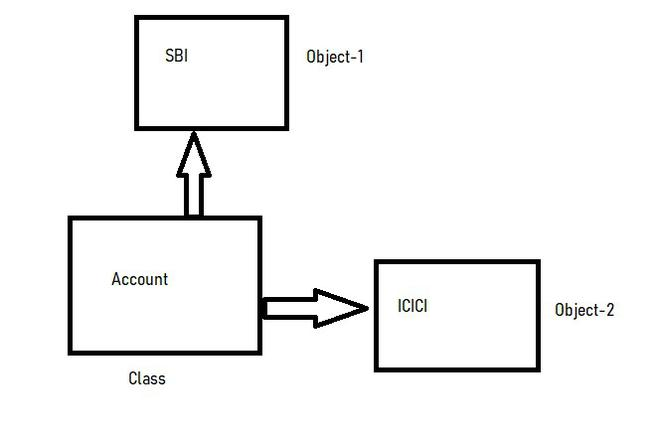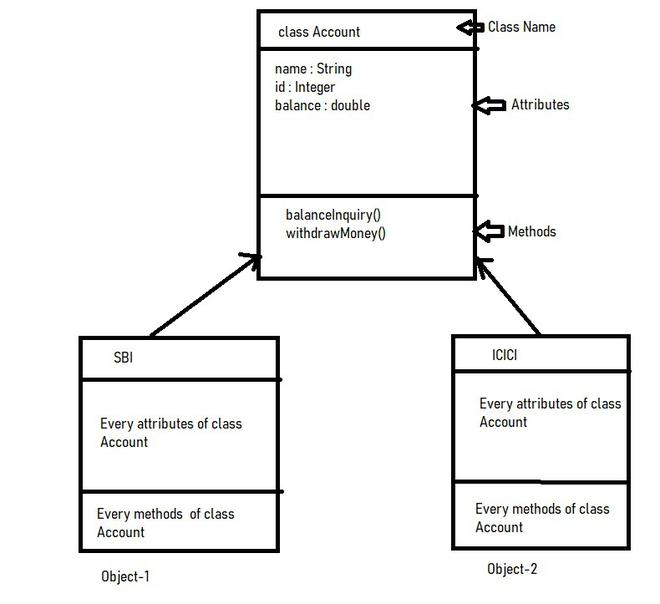# Difference Between Object And Class

• Difficulty Level : Easy
• Last Updated : 25 Aug, 2022

Class is a detailed description, the definition, and the template of what an object will be. But it is not the object itself. Also, what we call, a class is the building block that leads to Object-Oriented Programming. It is a user-defined data type, that holds its own data members and member functions, which can be accessed and used by creating an instance of that class. It is the blueprint of any object. Once we have written a class and defined it, we can use it to create as many objects based on that class as we want. In Java, the class contains fields, constructors, and methods. For example, consider the Class of Accounts. There may be many accounts with different names and types,  but all of them will share some common properties, as all of them will have some common attributes like balance, account holder name, etc. So here, the Account is the class.

Object is an instance of a class. All data members and member functions of the class can be accessed with the help of objects. When a class is defined, no memory is allocated, but memory is allocated when it is instantiated (i.e. an object is created). For Example, considering the objects for the class Account are SBI Account, ICICI account, etc.Fig-1: Pic Descriptions Class and objectFig-2: Class Diagram To Understand Class and Object

## Java

 `/*`` ``* Demo of class and object.`` ``* Example: Bank Account`` ``*/` `//Step-1 : Define Class Account``class` `Account{``    ``//Step-2 : Declare instance variable or fields and make it privates``    ``private` `String name;``    ``private` `int` `id;``    ``private` `double` `balance;``    ``private` `double` `money;``    ` `    ``//Step-3 : Define Non-Parameterized Constructor``    ``public` `Account() {``    ` `    ``}``    ``//Step-4 : Define Parameterized Constructor``    ``public` `Account(String name, ``int` `id, ``double` `balance) {``        ` `        ``this``.name = name;``        ``this``.id = id;``        ``this``.balance = balance;``    ``}``    ` `    ``//Step-5: Generate getter and setter``    ``public` `String getName() {``        ``return` `name;``    ``}``    ``public` `void` `setName(String name) {``        ``this``.name = name;``    ``}``    ``public` `int` `getId() {``        ``return` `id;``    ``}``    ``public` `void` `setId(``int` `id) {``        ``this``.id = id;``    ``}``    ``public` `double` `getBalance() {``        ``return` `balance;``    ``}``    ``public` `void` `setBalance(``double` `balance) {``        ``this``.balance = balance;``    ``}``    ``public` `double` `getMoney() {``        ``return` `money;``    ``}``    ``public` `void` `setMoney(``double` `money) {``        ``this``.money = money;``    ``}``    ``//Step-6: Generate toString() method``    ``@Override``    ``public` `String toString() {``        ``return` `"Account [name="` `+ name + ``", id="` `+ id + ``", balance="` `+ balance + ``"]"``;``    ``}``    ` `    ``//Step-7 : Add user-defined method-> balanceInquery()   ``    ``public` `void` `balanceInquery() {``        ``System.out.println( name + ``" Current Balance Is :: "` `+ balance );``    ``}``   ` `    ` `    ``//Step-8 : Add user-defined method-> withdrawMoney()``    ``public` `String  withdrawMoney() {``        ``return` `name + ``" Withdraw Money Successfully"``;``    ``}``    ` `}` `public` `class` `AccountType {` `    ``public` `static` `void` `main(String[] args) {``     ``//Step-9: Instantiate Objects Of class Account``        ``Account SBI = ``new` `Account(``"Raghab"``,``2211``,``70000.00``);``        ``Account ICICI = ``new` `Account(``"Navi"``,``1001``,``90000.00``);``        ` `    ``//Step-10: Access Attributes And Methods Of Class Account``    ` `    ``//For Account SBI ::``     ``System.out.println(SBI.toString());  ``//Access toString Method``     ``SBI.balanceInquery();    ``     ``SBI.setMoney(``5000``); ``//Set money Raghab wants to withdraw``    ` `     ``System.out.println(``"Raghab Withdraw Money From SBI:: "` `+ SBI.getMoney());``     ``System.out.println(``"Raghab Withdraw Money From SBI:: "` `+ SBI.withdrawMoney());``     ``System.out.println(``"----------------------------------------------------------"``);``    ` `    ``//For Account ICICI ::``     ``System.out.println(ICICI.toString());   ``//Access toString Method``     ``ICICI.balanceInquery();   ``     ``ICICI.setMoney(``1000``); ``//Set money Navi want to withdraw``     ` `     ``System.out.println(``"Navi Withdraw Money From ICICI:: "` `+ ICICI.getMoney());``     ``System.out.println(``"Navi Withdraw Money From ICICI:: "` `+ ICICI.withdrawMoney());``     ``System.out.println(``"----------------------------------------------------------"``);` `    ``}` `}`

## C#

 `// Include namespace system``using` `System;`  `// * Demo of class and object.``// * Example: Bank Account``// Step-1 : Define Class Account``public` `class` `Account``{``    ``// Step-2 : Declare instance variable or fields and make it privates``    ``private` `String name;``    ``private` `int` `id;``    ``private` `double` `balance;``    ``private` `double` `money;``    ``// Step-3 : Define Non-Parameterized Constructor``    ``// Step-4 : Define Parameterized Constructor``    ``public` `Account(String name, ``int` `id, ``double` `balance)``    ``{``        ``this``.name = name;``        ``this``.id = id;``        ``this``.balance = balance;``    ``}``    ``// Step-5: Generate getter and setter``    ``public` `String getName()``    ``{``        ``return` `this``.name;``    ``}``    ``public` `void` `setName(String name)``    ``{``        ``this``.name = name;``    ``}``    ``public` `int` `getId()``    ``{``        ``return` `this``.id;``    ``}``    ``public` `void` `setId(``int` `id)``    ``{``        ``this``.id = id;``    ``}``    ``public` `double` `getBalance()``    ``{``        ``return` `this``.balance;``    ``}``    ``public` `void` `setBalance(``double` `balance)``    ``{``        ``this``.balance = balance;``    ``}``    ``public` `double` `getMoney()``    ``{``        ``return` `this``.money;``    ``}``    ``public` `void` `setMoney(``double` `money)``    ``{``        ``this``.money = money;``    ``}``    ``// Step-6: Generate toString() method``    ``public` `String toString()``    ``{``        ``return` `"Account [name="` `+ ``this``.name + ``", id="` `+ ``this``.id.ToString() + ``", balance="` `+ ``this``.balance.ToString() + ``"]"``;``    ``}``    ``// Step-7 : Add user-defined method-> balanceInquery()``    ``public` `void` `balanceInquery()``    ``{``        ``Console.WriteLine(``this``.name + ``" Current Balance Is :: "` `+ ``this``.balance.ToString());``    ``}``    ``// Step-8 : Add user-defined method-> withdrawMoney()``    ``public` `String withdrawMoney()``    ``{``        ``return` `this``.name + ``" Withdraw Money Successfully"``;``    ``}``}``public` `class` `AccountType``{``    ``public` `static` `void` `Main(String[] args)``    ``{``        ``// Step-9: Instantiate Objects Of class Account``        ``var` `SBI = ``new` `Account(``"Raghab"``, 2211, 70000.0);``        ``var` `ICICI = ``new` `Account(``"Navi"``, 1001, 90000.0);``        ``// Step-10: Access Attributes And Methods Of Class Account``        ``// For Account SBI ::``        ``Console.WriteLine(SBI.toString());``        ``// Access toString Method``        ``SBI.balanceInquery();``        ``SBI.setMoney(5000);``        ``// Set money Raghab wants to withdraw``        ``Console.WriteLine(``"Raghab Withdraw Money From SBI:: "` `+ SBI.getMoney().ToString());``        ``Console.WriteLine(``"Raghab Withdraw Money From SBI:: "` `+ SBI.withdrawMoney());``        ``Console.WriteLine(``"----------------------------------------------------------"``);``        ``// For Account ICICI ::``        ``Console.WriteLine(ICICI.toString());``        ``// Access toString Method``        ``ICICI.balanceInquery();``        ``ICICI.setMoney(1000);``        ``// Set money Navi want to withdraw``        ``Console.WriteLine(``"Navi Withdraw Money From ICICI:: "` `+ ICICI.getMoney().ToString());``        ``Console.WriteLine(``"Navi Withdraw Money From ICICI:: "` `+ ICICI.withdrawMoney());``        ``Console.WriteLine(``"----------------------------------------------------------"``);``    ``}``}`

## Difference Between Class And Object:

There are many differences between object and class. Some differences between object and class are given below:

My Personal Notes arrow_drop_up